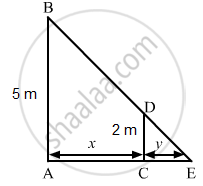Share

# A Man 2 Metres Tall Walks Away from a Lamp Post 5 Metres Height at the Rate of 4.8 Km/Hr. the Rate of Increase of the Length of His Shadow is (A) 1.6 Km/Hr (B) 6.3 Km/Hr(C) 5 Km/Hr (D) 3.2 Km/Hr - Mathematics

#### Question

A man 2 metres tall walks away from a lamp post 5 metres height at the rate of 4.8 km/hr. The rate of increase of the length of his shadow is

• 1.6 km/hr

• 6.3 km/hr

•  5 km/hr

• 3.2 km/hr

#### Solution

Let AB be the lamp post. Suppose at any time t, the man CD be at a distance of x km from the lamp post and y m be the length of his shadow CE.$\text { Since triangles ABE and CDE are similar },$

$\frac{AB}{CD} = \frac{AE}{CE}$

$\Rightarrow \frac{5}{2} = \frac{x + y}{y}$

$\Rightarrow \frac{x}{y} = \frac{5}{2} - 1$

$\Rightarrow \frac{x}{y} = \frac{3}{2}$

$\Rightarrow y = \frac{2}{3}x$

$\Rightarrow \frac{dy}{dt} = \frac{2}{3}\left( \frac{dx}{dt} \right)$

$\Rightarrow \frac{dy}{dt} = \frac{2}{3} \times 4 . 8$

$\Rightarrow \frac{dy}{dt} = 3 . 2 \ km/hr$

Is there an error in this question or solution?